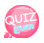# Recursive function

### Recursive functions can be difficult to look at words, which means calling your own function inside a function.

In other words, if you create a function called add, you call the add function inside the add function.
Once again,

```function add(){
}```

Same as above. ^^
This example uses the factorial in mathematics as an example.
There are some people who have a headache because of sudden math.
I can't see this course because I don't know what factorial is. Some people may have to go out.
Do not worry.

### What is factorial? You multiply your own number by one small number, then multiply by one small number, and multiply by one small number until it becomes 1. Let's say a number is 5, for example. The explanation can be weird, so give an example. 5x4x3x2x1. This is called factorial (!). The symbol is! is.

5! If you write like this and solve 5 !, 5 * 4 * 3 * 2 * 1, so 120 is the answer.
This is how we learned what factorial is.
Then 10! What is this?
10 * 9 * 8 * 7 * 6 * 5 * 4 * 3 * 2 * 1 ^-^ * The answer is 4,939,200.
So let's look at a recursive function that calls its own function inside a function.
By the way, this recursive function is a bit confusing if I understand and explain properly, but it is hard to find the data with the flow chart.
The source is:

### Let's understand the recursive function from the source below.

```function factorial(fnum){
end_num = 1;
if(fnum == end_num) return end_num;
else return fnum*factorial(fnum-1);
}
document.write(factorial(3));```

#### Function call

`document.write(factorial(3));`

The function call is sending parameter 3 with the output statement.

#### The factroial function takes 3 parameters.

```function factorial(fnum){
end_num = 1;
if(fnum == end_num) return end_num;
else return fnum*factorial(fnum-1);
}
document.write(factorial(3));```

fnum, a parameter of factroial, has a value of 3. Therefore, fnum = 3.

#### The reason why end_num = 1 is

`end_num = 1;`

Factorial multiplies until its number is one. Therefore, because end number is 1, declare end_num = 1.

#### If fnum and end_num have the same value

`if(fnum == end_num) return end_num;`

The end_num value is 1 and fnum is 3. If fnum is 1, end_num is returned.

#### if fnum is not equal to end_num

`else return fnum*factorial(fnum-1);`

Returns fnum * factroial (fnum-1). If fnum is 3,
Is to return 3 * factorial (2). So this part will go to the function call part?
However, since the value is passed but there is a call statement, factroial (2), the function is called to make 2 the value of the parameter, meets the else statement, and returns 2 * factorial (1).
If so, the factorial (3) statement is 3 * 2 * factorial (1). factorial (1) is called to return 1 because it is equal to end_num
The call statement will receive 3 * 2 * 1 as output. Do you understand? ^^
I don't think so but I'll explain it again.
Execute function call factorial (3)
fnum becomes 3 and 3 * factorial (2) is returned by else statement.
In 3 * factorial (2), factorial (2) is a function call and the parameter value is 2, so it meets the else statement and returns 2 * factrorial (1) and calls 1 as the parameter value.
Because end_num and fnum match, it meets the if statement and returns 1,
The function will return 3 * 2 * 1. ^^

```<!DOCTYPE html>
<html>
<meta charset="utf-8" />
<title>JavaScript</title>
<script>
function factorial(fnum){
end_num = 1;
if(fnum==end_num) return end_num;
else return fnum*factorial(fnum-1);
}
document.write("!="+factorial(3));
</script>